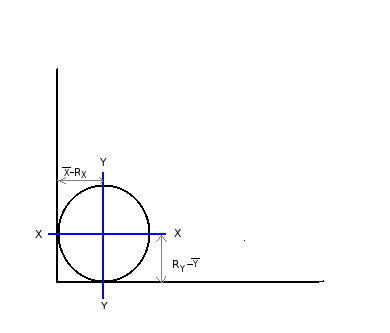# Moment of inertia for circle through centroid along x-x & y-y axes

Q.  Which of the following laminas have same moment of inertia (Ixx = Iyy), when passed through the centroid along x-x and y-y axes?
- Published on 21 Sep 15

a. Circle
b. Semi-circle
c. Right angle triangle
d. Isosceles triangle

#### Discussion

• Sravanthi   -Posted on 24 Nov 15- Moment of inertia along Ixx = (π/64) D4

- Moment of inertia along Iyy = (π/64) D4

- For semi-circle

Ixx = 0.11 R4

Iyy = (π/128) D4 or (π/8) R4

- For right angled triangle

Ixx =Iyy = bh3/36

- Isosceles triangle

- Moment of inertia along Ixx = bh3/ 36

- Moment of inertia along Iyy = hb3/ 48

## ➨ Post your comment / Share knowledgeEnter the code shown above:

(Note: If you cannot read the numbers in the above image, reload the page to generate a new one.)